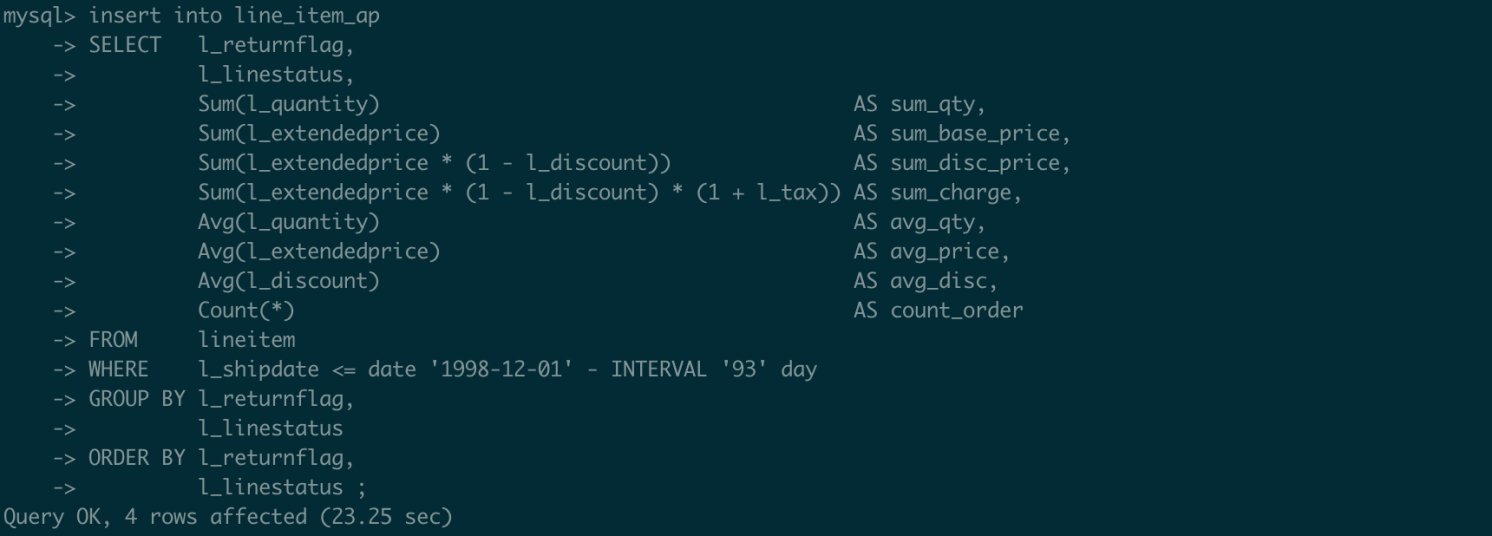TPC Benchmark H (TPC-H) queries are used as examples in this topic to describe how to use parallel queries.

## Test design

• Data volume: The data volume for testing is 100 GB. The scale factor is 100.
• ApsaraDB PolarDB MySQL-compatible edition 8.0 cluster: The node specification is 88 CPU cores and 710 GB memory. The test is performed on the primary node of the cluster.

## Support for GROUP BY and ORDER BY

The following code block shows an example of the original SQL statement:

``````SELECT   l_returnflag,
l_linestatus,
Sum(l_quantity)                                       AS sum_qty,
Sum(l_extendedprice)                                  AS sum_base_price,
Sum(l_extendedprice * (1 - l_discount))               AS sum_disc_price,
Sum(l_extendedprice * (1 - l_discount) * (1 + l_tax)) AS sum_charge,
Avg(l_quantity)                                       AS avg_qty,
Avg(l_extendedprice)                                  AS avg_price,
Avg(l_discount)                                       AS avg_disc,
Count(*)                                              AS count_order
FROM     lineitem
WHERE    l_shipdate <= date '1998-12-01' - INTERVAL '93' day
GROUP BY l_returnflag,
l_linestatus
ORDER BY l_returnflag,
l_linestatus ;``````
• Before the parallel query feature is enabled, it takes 1,563.32 seconds to run the query.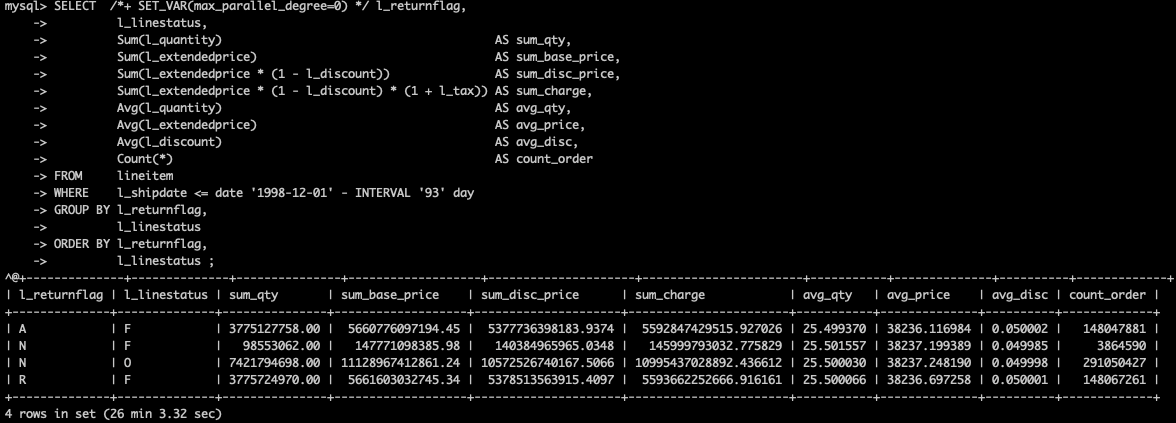• After the parallel query feature is enabled, it takes only 49.65 seconds to run the query. The consumed time is 31.48 times shorter than the original query time.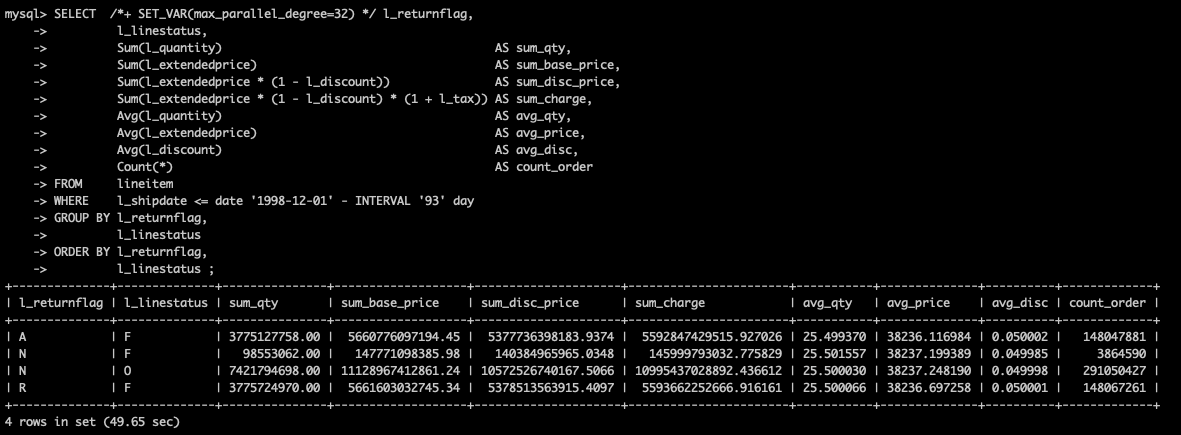## Support for AGGREGATE functions (SUM, AVG, and COUNT)

The following code block shows an example of the original SQL statement:

``````SELECT   l_returnflag,
l_linestatus,
Sum(l_quantity)                                       AS sum_qty,
Sum(l_extendedprice)                                  AS sum_base_price,
Sum(l_extendedprice * (1 - l_discount))               AS sum_disc_price,
Sum(l_extendedprice * (1 - l_discount) * (1 + l_tax)) AS sum_charge,
Avg(l_quantity)                                       AS avg_qty,
Avg(l_extendedprice)                                  AS avg_price,
Avg(l_discount)                                       AS avg_disc,
Count(*)                                              AS count_order
FROM     lineitem
WHERE    l_shipdate <= date '1998-12-01' - INTERVAL '93' day
GROUP BY l_returnflag,
l_linestatus
ORDER BY l_returnflag,
l_linestatus ;``````
• Before the parallel query feature is enabled, it takes 1,563.32 seconds to run the query.• After the parallel query feature is enabled, it takes only 49.65 seconds to run the query. The consumed time is 31.48 times shorter than the original query time.## Support for JOIN

The following code block shows an example of the original SQL statement:

``````select sum(l_extendedprice* (1 - l_discount)) as revenue
from   lineitem,   part
where ( p_partkey = l_partkey and p_brand = 'Brand#12'
and p_container in ('SM CASE', 'SM BOX', 'SM PACK', 'SM PKG')
and l_quantity >= 6 and l_quantity <= 6 + 10
and p_size between 1 and 5
and l_shipmode in ('AIR', 'AIR REG')
and l_shipinstruct = 'DELIVER IN PERSON' )
or ( p_partkey = l_partkey and p_brand = 'Brand#13'
and p_container in ('MED BAG', 'MED BOX', 'MED PKG', 'MED PACK')
and l_quantity >= 10 and l_quantity <= 10 + 10
and p_size between 1 and 10
and l_shipmode in ('AIR', 'AIR REG')
and l_shipinstruct = 'DELIVER IN PERSON' )
or ( p_partkey = l_partkey and p_brand = 'Brand#24'
and p_container in ('LG CASE', 'LG BOX', 'LG PACK', 'LG PKG')
and l_quantity >= 21 and l_quantity <= 21 + 10
and p_size between 1 and 15
and l_shipmode in ('AIR', 'AIR REG')
and l_shipinstruct = 'DELIVER IN PERSON' ); ``````
• Before the parallel query feature is enabled, it takes 21.73 seconds to run the query.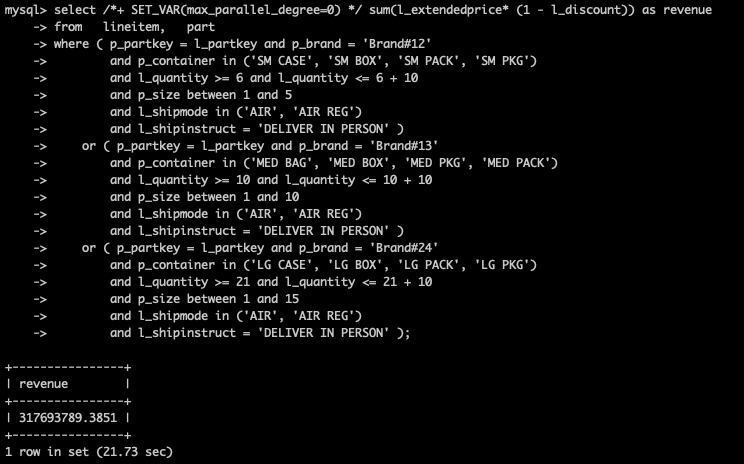• After the parallel query feature is enabled, it takes only 1.37 seconds to run the query. The consumed time is 15.86 times shorter than the original query time.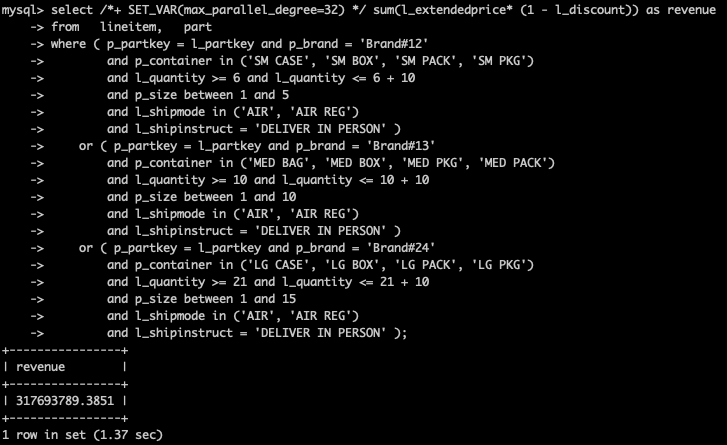## Support for BETWEEN and IN functions

The following code block shows an example of the original SQL statement:

``````select sum(l_extendedprice* (1 - l_discount)) as revenue
from   lineitem,   part
where ( p_partkey = l_partkey and p_brand = 'Brand#12'
and p_container in ('SM CASE', 'SM BOX', 'SM PACK', 'SM PKG')
and l_quantity >= 6 and l_quantity <= 6 + 10
and p_size between 1 and 5
and l_shipmode in ('AIR', 'AIR REG')
and l_shipinstruct = 'DELIVER IN PERSON' )
or ( p_partkey = l_partkey and p_brand = 'Brand#13'
and p_container in ('MED BAG', 'MED BOX', 'MED PKG', 'MED PACK')
and l_quantity >= 10 and l_quantity <= 10 + 10
and p_size between 1 and 10
and l_shipmode in ('AIR', 'AIR REG')
and l_shipinstruct = 'DELIVER IN PERSON' )
or ( p_partkey = l_partkey and p_brand = 'Brand#24'
and p_container in ('LG CASE', 'LG BOX', 'LG PACK', 'LG PKG')
and l_quantity >= 21 and l_quantity <= 21 + 10
and p_size between 1 and 15
and l_shipmode in ('AIR', 'AIR REG')
and l_shipinstruct = 'DELIVER IN PERSON' ); ``````
• Before the parallel query feature is enabled, it takes 21.73 seconds to run the query.• After the parallel query feature is enabled, it takes only 1.37 seconds to run the query. The consumed time is 15.86 times shorter than the original query time.## Support for LIMIT

The following code block shows an example of the original SQL statement:

``````select l_shipmode, sum(case when o_orderpriority = '1-URGENT' or o_orderpriority = '2-HIGH' then 1
else 0
end) as high_line_count, sum(case when o_orderpriority <> '1-URGENT' and o_orderpriority <> '2-HIGH' then 1
else 0
end) as low_line_count
from   orders,   lineitem
where o_orderkey = l_orderkey
and l_shipmode in ('MAIL', 'TRUCK')
and l_commitdate < l_receiptdate
and l_shipdate < l_commitdate
and l_receiptdate >= date '1996-01-01'
and l_receiptdate < date '1996-01-01' + interval '1' year
group by l_shipmode
order by l_shipmode limit 10; ``````
• Before the parallel query feature is enabled, it takes 339.22 seconds to run the query.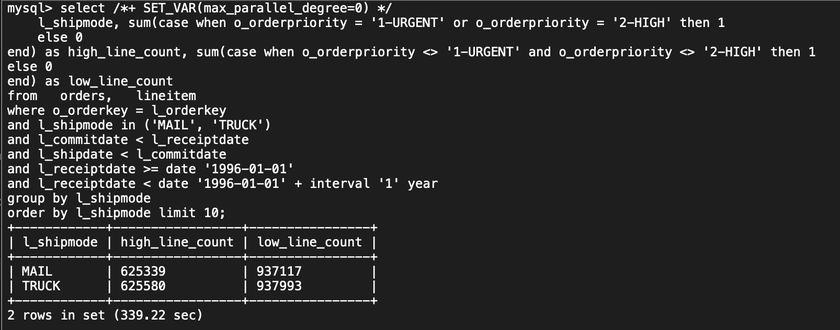• After the parallel query feature is enabled, it takes only 29.31 seconds to run the query. The consumed time is 11.57 times shorter than the original query time.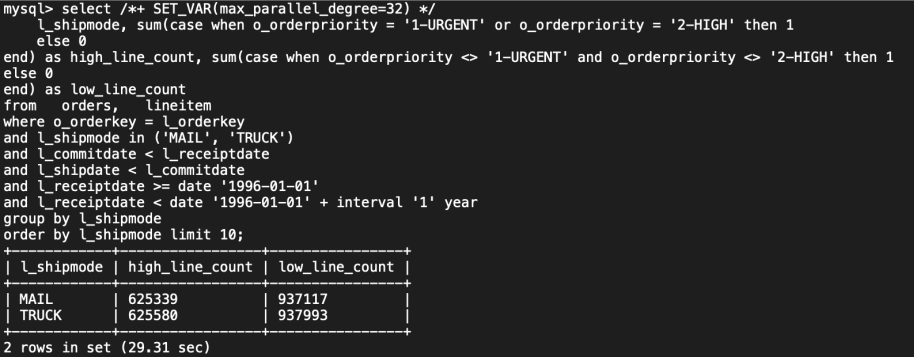## Support for INTERVAL functions

The following code block shows an example of the original SQL statement:

``````select
100.00 * sum(case when p_type like 'PROMO%' then l_extendedprice * (1 - l_discount)
else 0
end) / sum(l_extendedprice * (1 - l_discount)) as promo_revenue
from   lineitem,   part
where l_partkey = p_partkey
and l_shipdate >= date '1996-01-01'
and l_shipdate < date '1996-01-01' + interval '1' month limit 10; ``````
• Before the parallel query feature is enabled, it takes 220.87 seconds to run the query.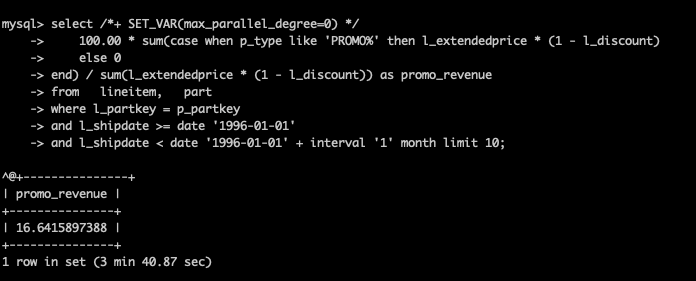• After the parallel query feature is enabled, it takes only 7.75 seconds to run the query. The consumed time is 28.5 times shorter than the original query time.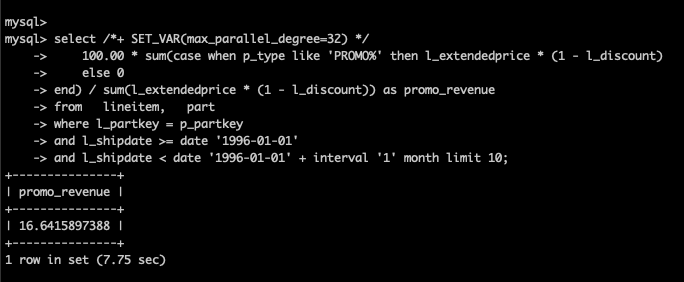## Support for CASE WHEN

The following code block shows an example of the original SQL statement:

``````select
100.00 * sum(case when p_type like 'PROMO%' then l_extendedprice * (1 - l_discount)
else 0
end) / sum(l_extendedprice * (1 - l_discount)) as promo_revenue
from   lineitem,   part
where l_partkey = p_partkey
and l_shipdate >= date '1996-01-01'
and l_shipdate < date '1996-01-01' + interval '1' month limit 10; ``````
• Before the parallel query feature is enabled, it takes 220.87 seconds to run the query.• After the parallel query feature is enabled, it takes only 7.75 seconds to run the query. The consumed time is 28.5 times shorter than the original query time.## Support for LIKE

The following code block shows an example of the original SQL statement:

``````select s_name, s_address from
supplier,  nation where
s_suppkey in
( select ps_suppkey from  partsupp where
ps_partkey in ( select p_partkey from  part where p_name like 'dark%')
and ps_availqty>(select 0.0005 * sum(l_quantity) as col1
from   lineitem,   partsupp
where l_partkey = ps_partkey and l_suppkey = ps_suppkey
and l_shipdate >= date '1993-01-01' and l_shipdate < date '1993-01-01' + interval '1' year)
)
and s_nationkey = n_nationkey and n_name = 'JORDAN'
order by s_name limit 10; ``````
• Before the parallel query feature is enabled, it takes 427.46 seconds to run the query.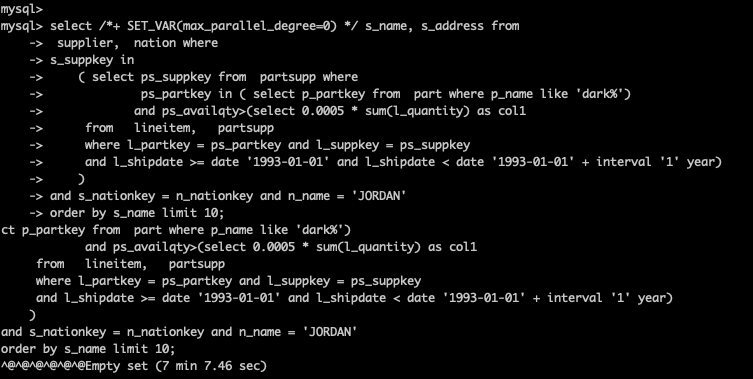• After the parallel query feature is enabled, it takes only 33.72 seconds to run the query. The consumed time is 12.68 times shorter than the original query time.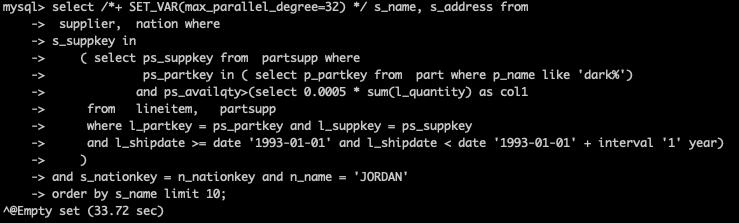## Support for subqueries

The following code block shows an example of the original SQL statement:

``````select
s_acctbal,
s_name,
n_name,
p_partkey,
p_mfgr,
s_phone,
s_comment
from
part,
supplier,
partsupp,
nation,
region
where
p_partkey = ps_partkey
and s_suppkey = ps_suppkey
and p_size = 35
and p_type like '%STEEL'
and s_nationkey = n_nationkey
and n_regionkey = r_regionkey
and r_name = 'AMERICA'
and ps_supplycost = (
select
min(ps_supplycost)
from
partsupp,
supplier,
nation,
region
where
p_partkey = ps_partkey
and s_suppkey = ps_suppkey
and s_nationkey = n_nationkey
and n_regionkey = r_regionkey
and r_name = 'AMERICA'
)
order by
s_acctbal desc,
n_name,
s_name,
p_partkey;
limit 100;``````
• Before the parallel query feature is enabled, it takes 16.23 seconds to run the query.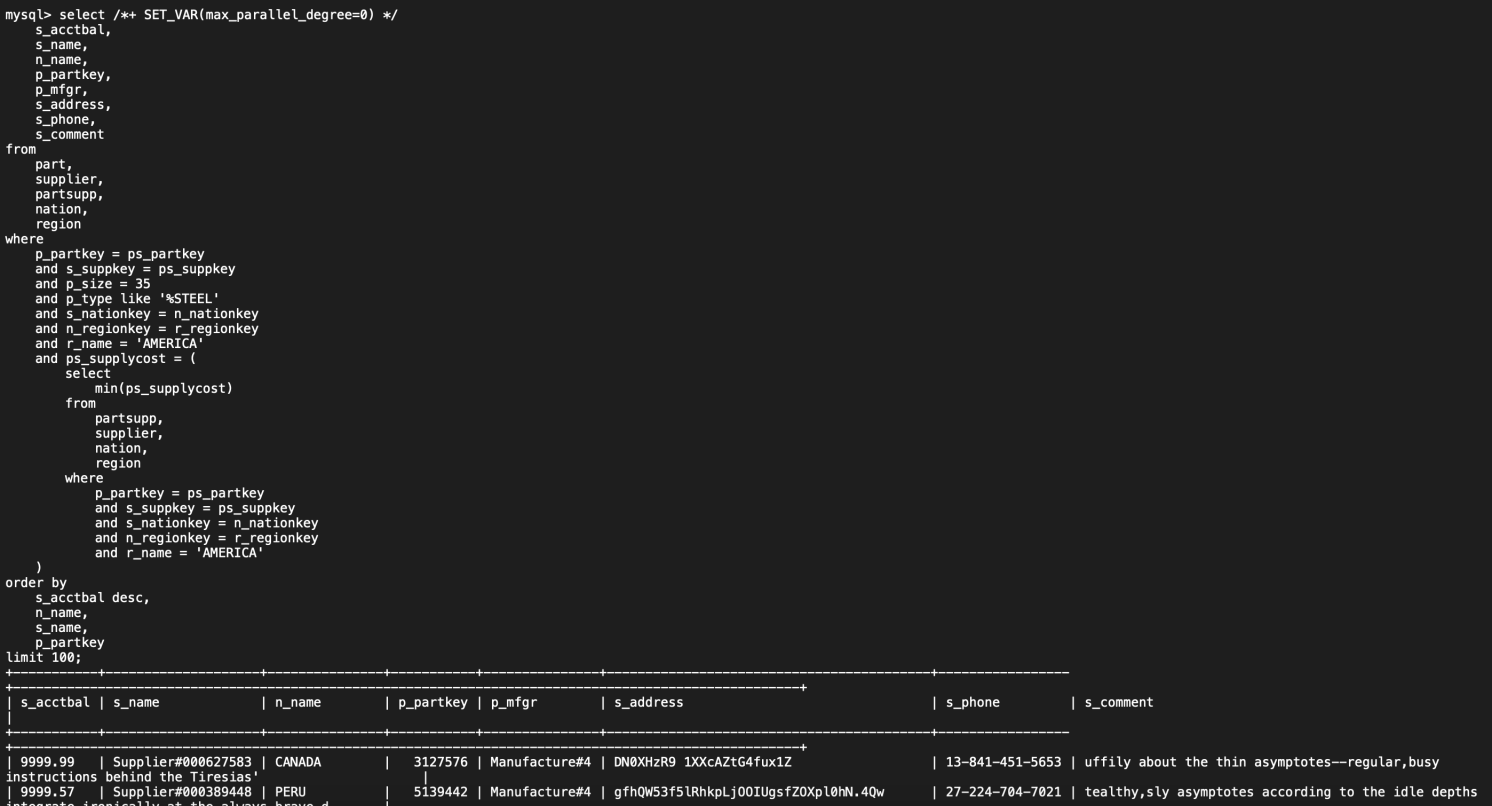• After the parallel query feature is enabled, it takes only 1.4 seconds to run the query. The consumed time is 11.59 times shorter than the original query time.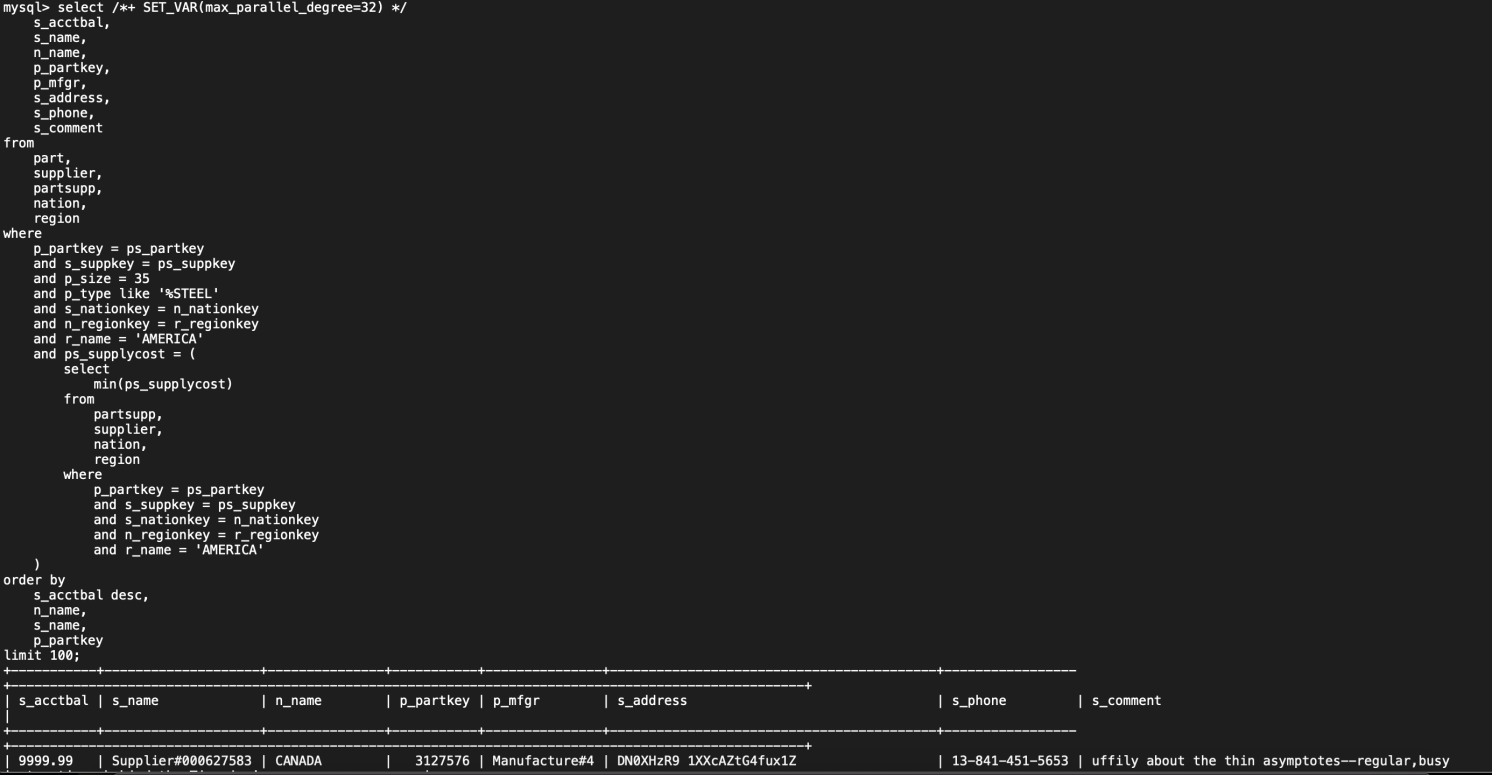## Support for GROUP BY WITH ROLLUP

The following code block shows an example of the original SQL statement:

``````select
l_returnflag,
l_linestatus,
sum(l_quantity) as sum_qty,
sum(l_extendedprice) as sum_base_price,
sum(l_extendedprice * (1 - l_discount)) as sum_disc_price,
sum(l_extendedprice * (1 - l_discount) * (1 + l_tax)) as sum_charge,
avg(l_quantity) as avg_qty,
avg(l_extendedprice) as avg_price,
avg(l_discount) as avg_disc,
count(*) as count_order
from
lineitem
where
l_shipdate <= date_sub('1998-12-01', interval ':1' day)
group by
l_returnflag,
l_linestatus
with rollup
order by
l_returnflag,
l_linestatus;``````
• Before the parallel query feature is enabled, it takes 318.73 seconds to run the query.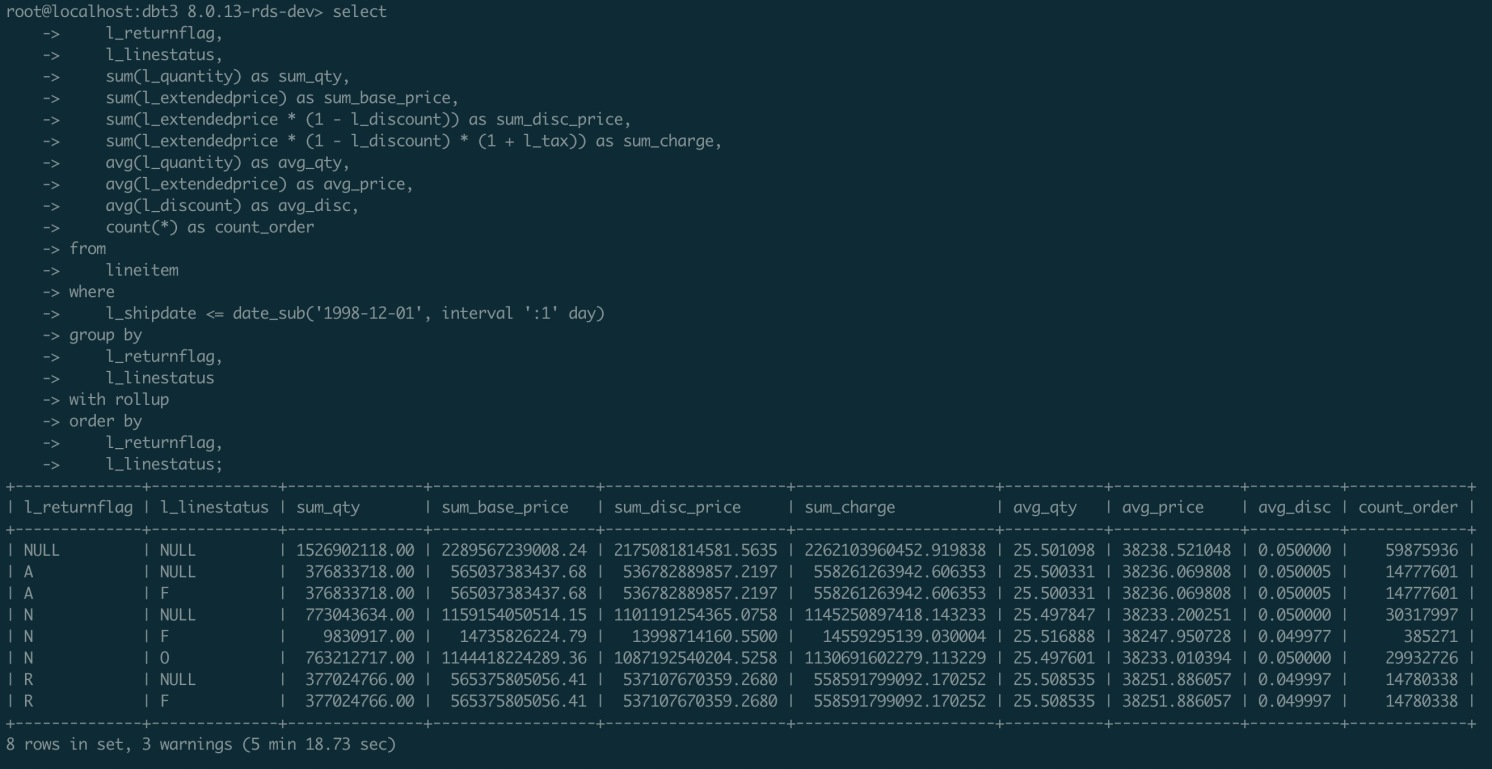• After the parallel query feature is enabled, it takes only 22.30 seconds to run the query. The consumed time is 14.29 times shorter than the original query time.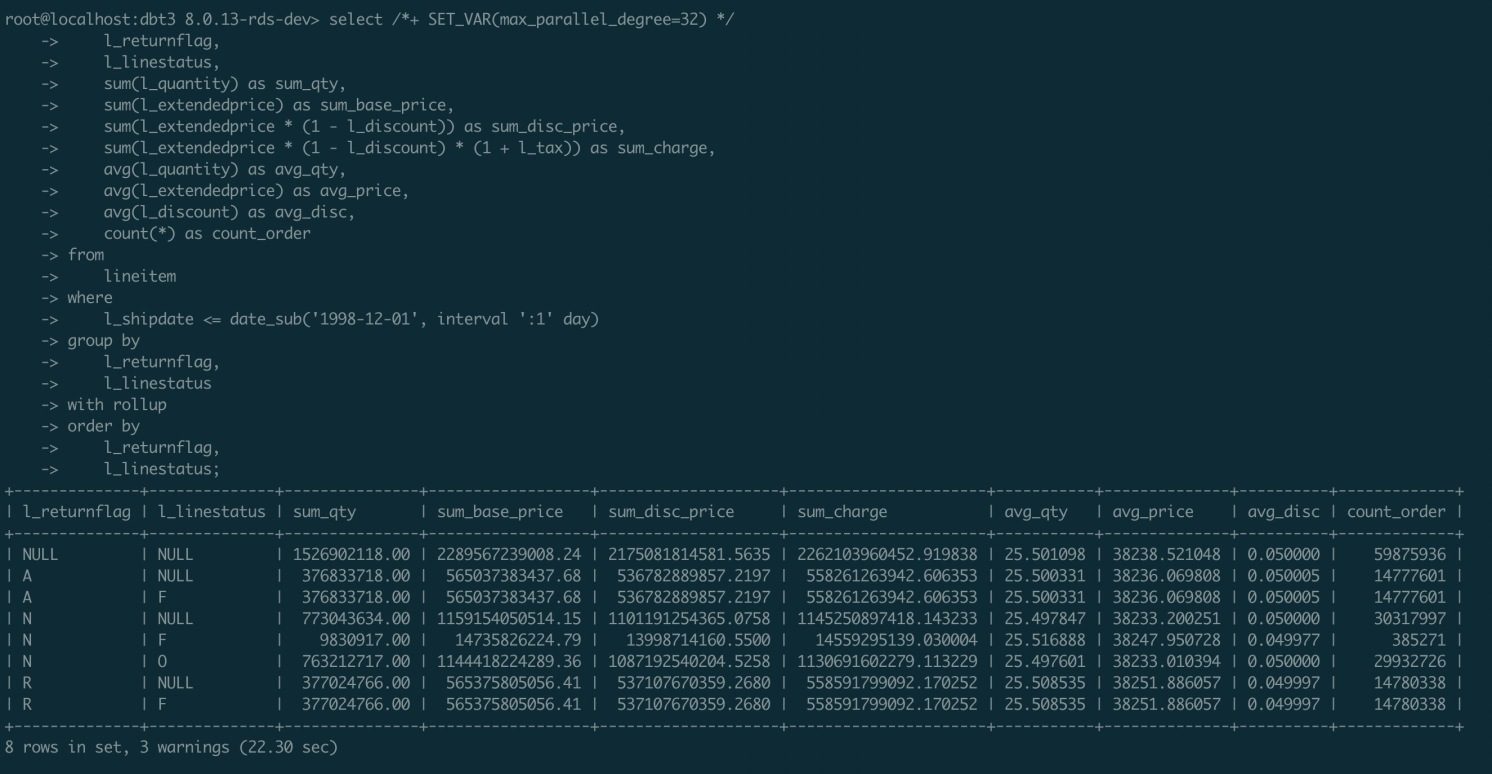## Support for INSERT ... SELECT and REPLACE ... SELECT

The following code block shows an example of the original SQL statement:

``````insert into line_item_ap
SELECT   l_returnflag,
l_linestatus,
Sum(l_quantity)                                       AS sum_qty,
Sum(l_extendedprice)                                  AS sum_base_price,
Sum(l_extendedprice * (1 - l_discount))               AS sum_disc_price,
Sum(l_extendedprice * (1 - l_discount) * (1 + l_tax)) AS sum_charge,
Avg(l_quantity)                                       AS avg_qty,
Avg(l_extendedprice)                                  AS avg_price,
Avg(l_discount)                                       AS avg_disc,
Count(*)                                              AS count_order
FROM     lineitem
WHERE    l_shipdate <= date '1998-12-01' - INTERVAL '93' day
GROUP BY l_returnflag,
l_linestatus
ORDER BY l_returnflag,
l_linestatus ;``````
• Before the parallel query feature is enabled, it takes 182.82 seconds to run the query.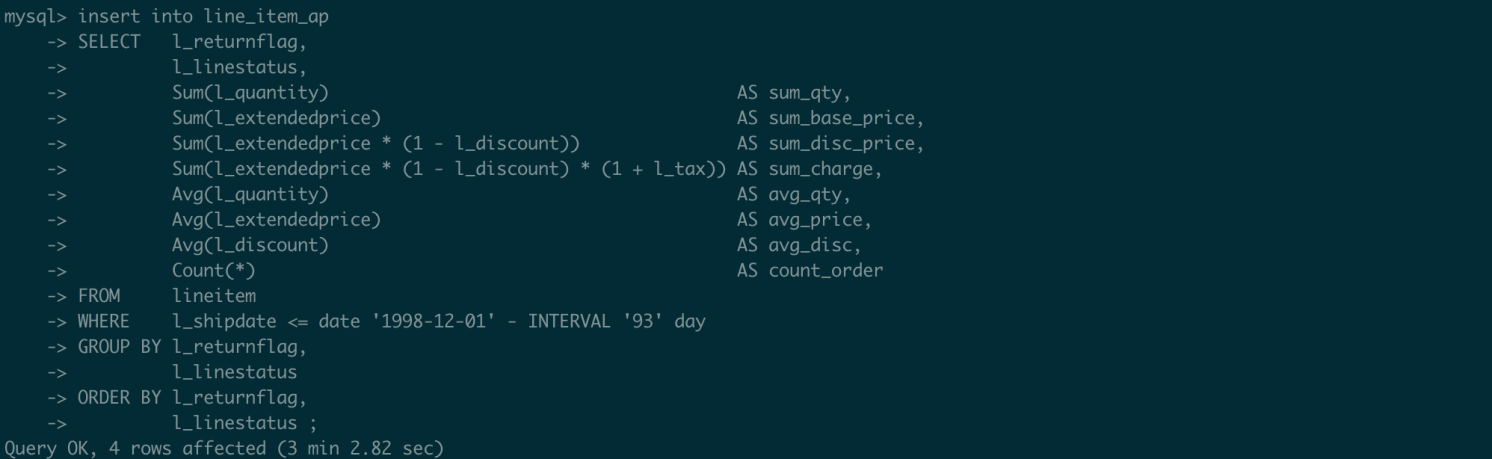• After the parallel query feature is enabled, it takes only 23.25 seconds to run the query. The consumed time is 7.86 times shorter than the original query time.